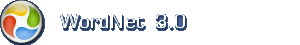English dictionary

Hint: Asterisk (*) is a wildcard. Asterisk substitutes zero or more characters.

English noun: mathematical relation

1. mathematical relation a relation between mathematical expressions (such as equality or inequality)

Narrower (hyponym)function, map, mapping, mathematical function, parity, reflexiveness, reflexivity, single-valued function, transitivity

Domain categorymath, mathematics, maths

Based on WordNet 3.0 copyright © Princeton University.
Web design: Orcapia v/Per Bang. English edition: .
2019 onlineordbog.dk